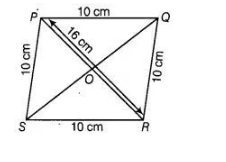# If the side of a rhombus is 10 cm and`
Question:

If the side of a rhombus is 10 cm and one diagonal is 16 cm, then area of the rhombus is 96 cm2.

Solution:

True

Given, side of a rhombus PQRS is 10 cm and one of the diagonal is 16 cm.

i.e., PQ = QR = RS =SP = 10 cm and PR = 16 cmIn ΔPOQ, PQ2 = OP2 + OQ2 [by Pythagoras theorem]

[ since, the diagonal of rhombus bisects each other at 90°] => OQ2 = PQ2 – OP2 = (10)2 – (8)2

=> OQ2 =100 – 64 = 36

=> OQ = 6 cm

[taking positive square root because length is always positive] SQ =2 x OP = 2 x 6= 12 cm

Area of the rhombus = ½ (Product of diagonals)

= ½ (OS x PR) =½ x 12 x 16 = 96 cm2.